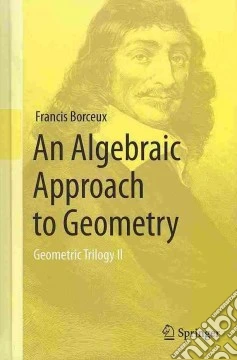ricerca
avanzata

# An Algebraic Approach to Geometry

## Un libro in lingua di Francis Borceux edito da Springer Verlag, 2013

• € 116,20
• Il prezzo è variabile in funzione del cambio della valuta d’origineThis is a unified treatment of the various algebraic approaches to geometric spaces. The study of algebraic curves in the complex projective plane is the natural link between linear geometry at an undergraduate level and algebraic geometry at a graduate level, and it is also an important topic in geometric applications, such as cryptography.

380 years ago, the work of Fermat and Descartes led us to study geometric problems using coordinates and equations. Today, this is the most popular way of handling geometrical problems. Linear algebra provides an efficient tool for studying all the first degree (lines, planes) and second degree (ellipses, hyperboloids) geometric figures, in the affine, the Euclidean, the Hermitian and the projective contexts. But recent applications of mathematics, like cryptography, need these notions not only in real or complex cases, but also in more general settings, like in spaces constructed on finite fields. And of course, why not also turn our attention to geometric figures of higher degrees? Besides all the linear aspects of geometry in their most general setting, this book also describes useful algebraic tools for studying curves of arbitrary degree and investigates results as advanced as the Bezout theorem, the Cramer paradox, topological group of a cubic, rational curves etc.

Hence the book is of interest for all those who have to teach or study linear geometry: affine, Euclidean, Hermitian, projective; it is also of great interest to those who do not want to restrict themselves to the undergraduate level of geometric figures of degree one or two.